Histogram

Chapter 5 Class 8 Data Handling
Concept wise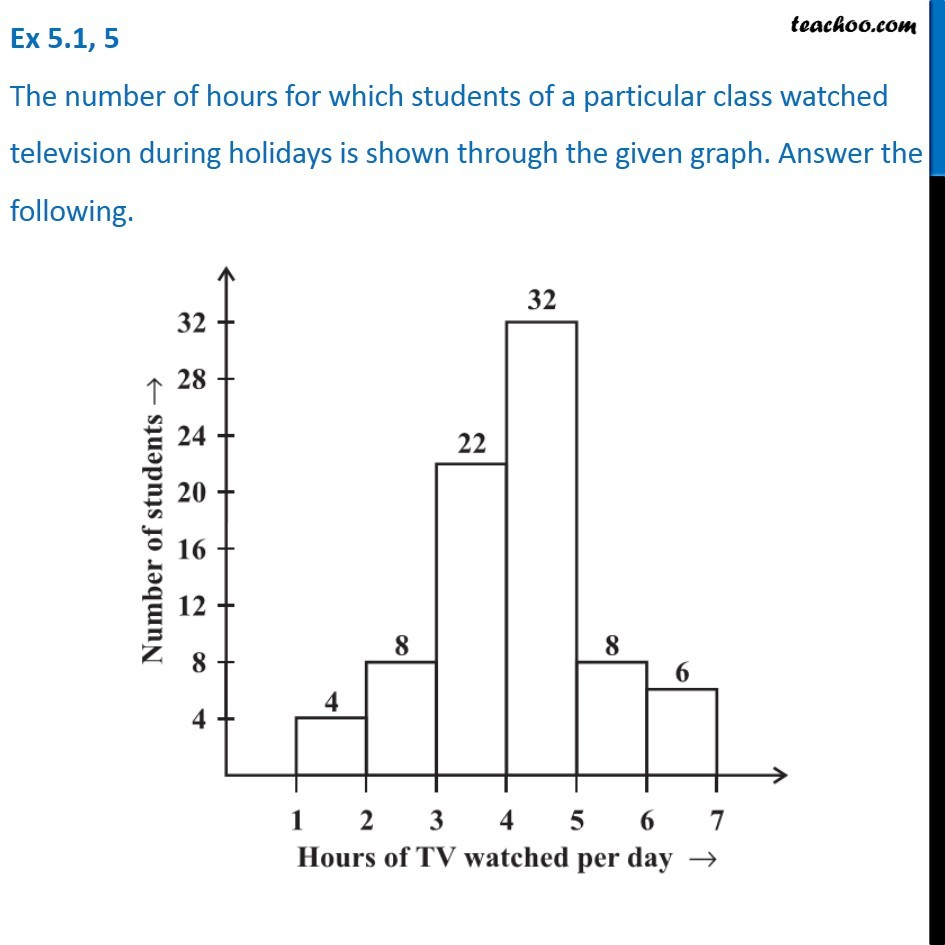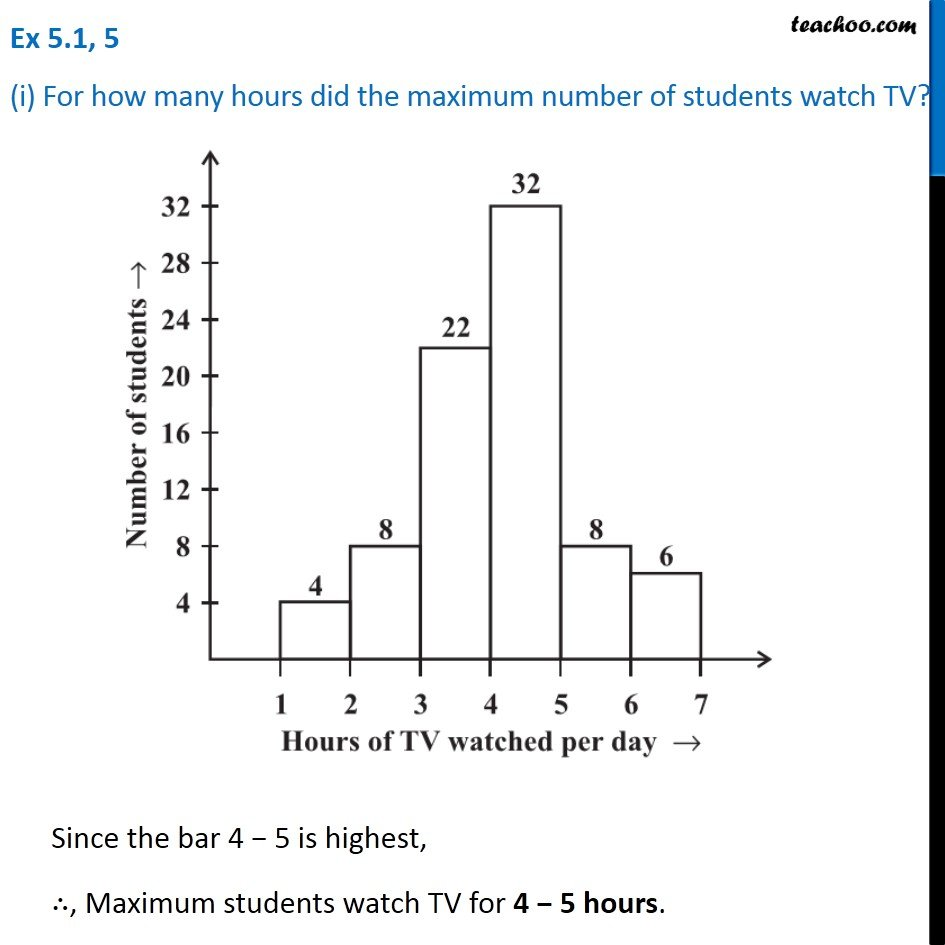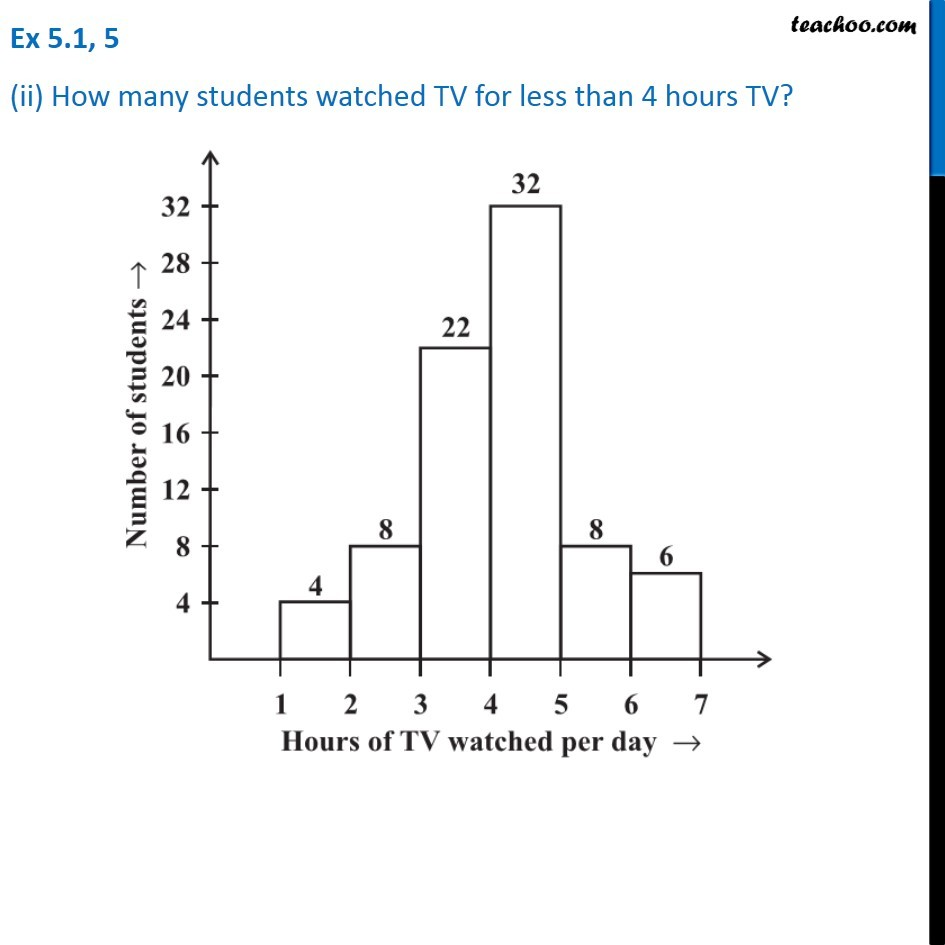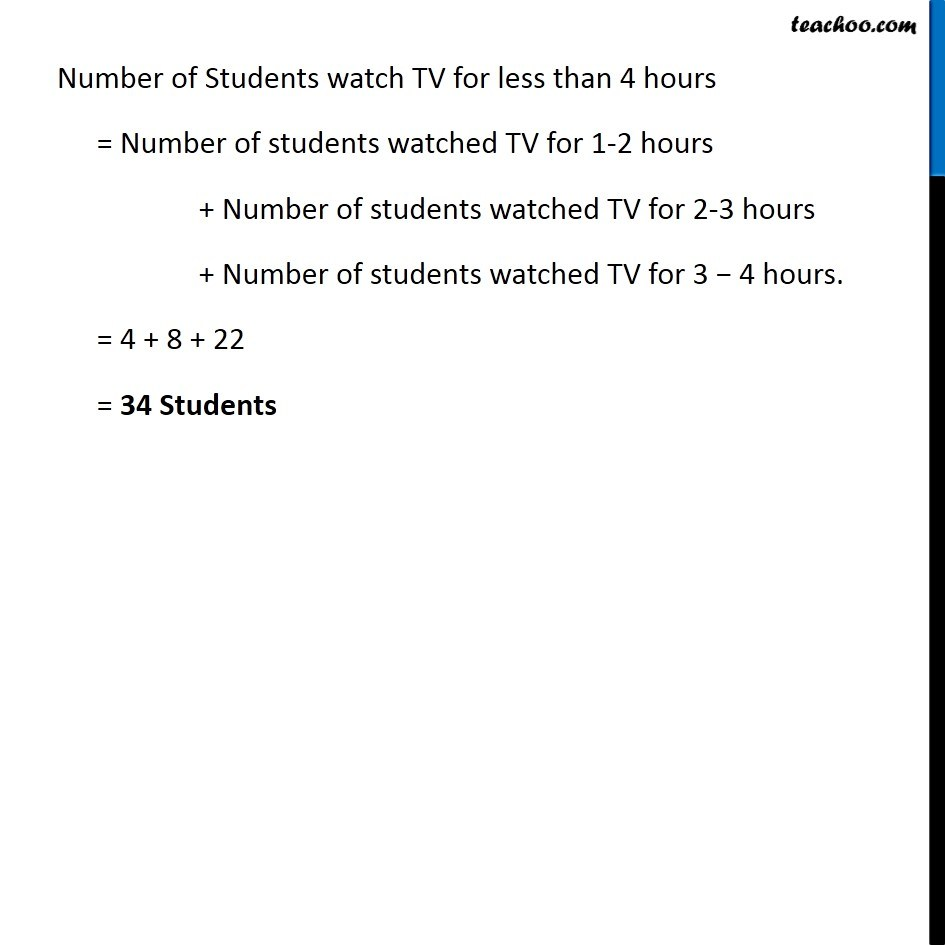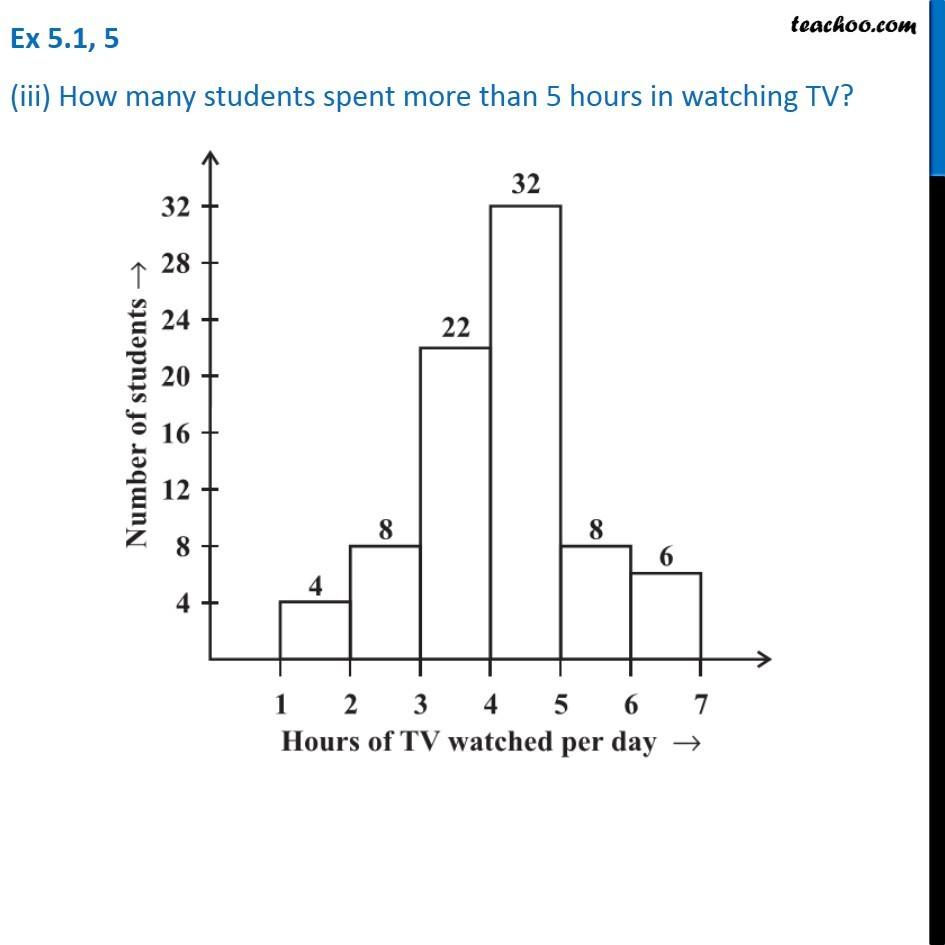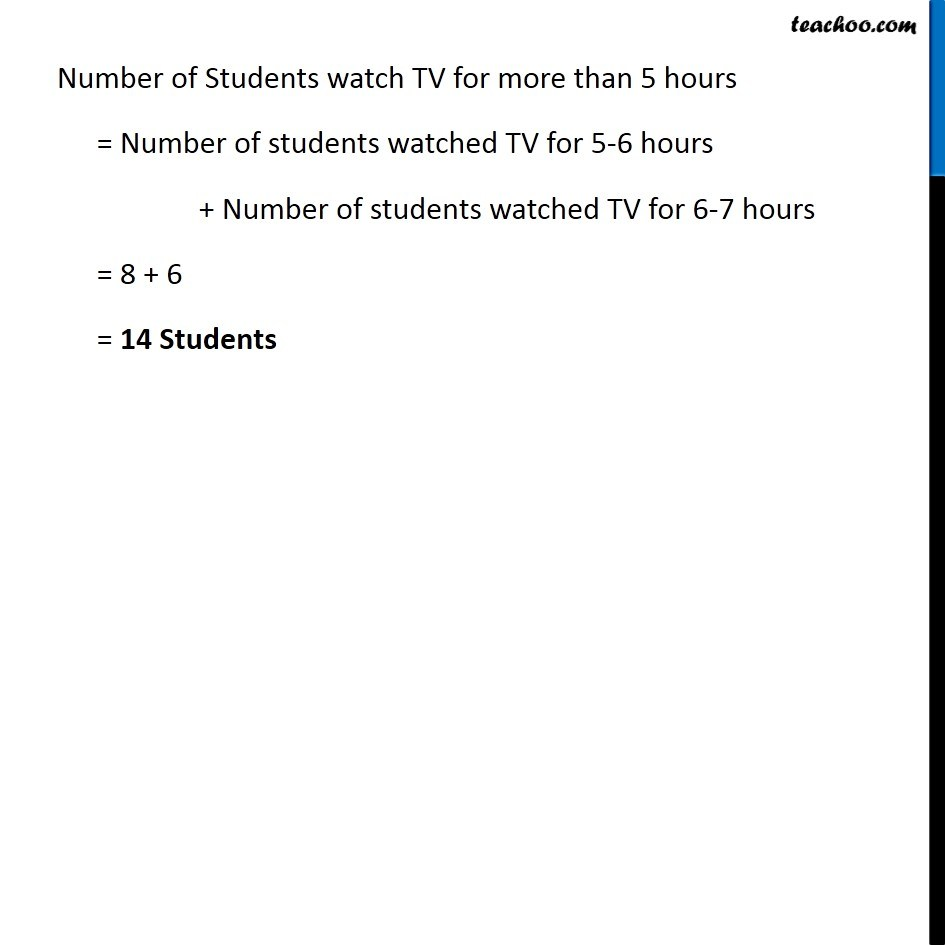Introducing your new favourite teacher - Teachoo Black, at only ₹83 per month

### Transcript

Ex 5.1, 5 The number of hours for which students of a particular class watched television during holidays is shown through the given graph. Answer the following. Ex 5.1, 5 (i) For how many hours did the maximum number of students watch TV? Since the bar 4 − 5 is highest, ∴, Maximum students watch TV for 4 − 5 hours. Ex 5.1, 5 (ii) How many students watched TV for less than 4 hours TV? Number of Students watch TV for less than 4 hours = Number of students watched TV for 1-2 hours + Number of students watched TV for 2-3 hours + Number of students watched TV for 3 − 4 hours. = 4 + 8 + 22 = 34 Students Ex 5.1, 5 (iii) How many students spent more than 5 hours in watching TV? Number of Students watch TV for more than 5 hours = Number of students watched TV for 5-6 hours + Number of students watched TV for 6-7 hours = 8 + 6 = 14 Students